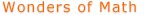Home    |    Teacher    |    Parents    |    Glossary    |    About UsEmail this page to a friendMore Wonders· Fractals · Spirograph · Conway's Game of Life · Roman Numeral Calculator · Chaos · Knots · Mazes · Tessellations · Oragami · Lissajous LabResources· Cool Tools · Formulas & Tables · References · Test Preparation · Study Tips · Wonders of MathSearchSpirograph

You will need a Java capable browser to see these graphics.

What is a Spirograph?
A Spirograph is formed by rolling a circle inside or outside of another circle. The pen is placed at any point on the rolling circle. If the radius of fixed circle is R, the radius of moving circle is r, and the offset of the pen point in the moving circle is O, then the equation of the resulting curve is defined by:

```

x = (R+r)*cos(t) - (r+O)*cos(((R+r)/r)*t)

y = (R+r)*sin(t) - (r+O)*sin(((R+r)/r)*t)

```
Here is how you can use the controls in this Spirograph applet:

• The first three scroll bars in the control panel let you change R, r and O respectively. You can change these values between 1 and 100.

• You can use the next three scroll bars to change the color of the drawing. These scroll bars change the red, green and blue values of the color (in the range 0-255) respectively.

• The last scroll bar lets you choose the number of iterations for the Spirograph.

• You can use the Random button to select random values for the radii and color. The number of iterations is not changed by the Random button.

Created by Anu Garg.

Other Wonders of Math

 Fractals Chaos Tessellations Spirograph Knots Origami Conway's Game of Life Mazes Lissajous Lab Roman Numeral Calculator